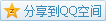# （二元）关系

## 前置要点 Highlights

P. 26 Para. 3: We may not know what a relation is, but we do know what a set is, and the preceding considerations establish a close connection between relations and sets.

• 使用集合语言，去描述关系.

## 阐明 Exposition

• 二元关系

• 由二元有序对组成的集合即是一个二元关系，也就是说$z\in R\Leftrightarrow \exists x,y:z=(x,y)$，那么$R$就是一个二元关系.
• 如果有序对$(x,y)\in R$，那么我们有时为了方便会写成$x\;R\;y$.
• 二元关系例举

• $R = \varnothing$是一个二元关系. (反面情况思考)
• $R = A\times B$是一个二元关系.
• $R=\{(x,x): x\in A\}$是一个在$A$上的相等关系$x\;R\;y$意味着$x = y$.
• 投影

• 将二元关系$R$第一维的投影称为定义域(domain)，记作$\mathrm{dom} R$，被定义为$\mathrm{dom} R = \{x:\exists y (x\;R\;y)\}$.
• 将二元关系$R$第二维的投影称为值域(range)，记作$\mathrm{ran}R$，被定义为$\mathrm{ran} R = \{y:\exists x (x\;R\;y)\}$.
• 投影的例举

• 考虑一个$X$$\mathcal{P}(X)$的属于关系$R=\{(a,b): a\in X, b\in \mathcal{P}(X)\}$，此处$\mathrm{dom} A= X$，而$\mathrm{ran} A=\mathcal{P}(X) - \{\varnothing\}$.
• 一些用语以及性质
• 如果$R$满足$\mathrm{dom} A\subset X, \mathrm{ran} A\subset Y$，那么称其为从$X$$Y$的一个关系.
• 如果$R$是从$X$$X$的一个关系，那么常称其为$X$上的关系.
• 如果$X$上的关系$R$满足$\forall x\in X, x\;R \;x$，那么$R$具有自反性(reflexive).
• 如果从$X$$Y$的关系$R$满足$\forall x\in X,\forall y\in Y, x\;R\;y \Leftrightarrow y\;R\;x$，那么$R$具有对称性(symmetric).
• 如果从$X$$Y$的关系$R$满足$\forall x,\forall y,\forall z, (x\;R\;y \land y\;R\;z) \Rightarrow x\;R\;z$，那么$R$具有传递性(transitive).

• 等价关系

• 如果关系$R$满足自反性、对称性、传递性，则其为等价关系.
• 对于$X$上的关系，最小的等价关系是$X$上的相等关系，最大的等价关系是笛卡儿积$X\times X$.
• 划分等价类

• 如果$P$$X$非空子集的集合，且$P$中的元素不交，$\bigcup P = X$，那么$P$$X$的一个划分.
• 如果$R$$X$上的一个等价关系，如果$x\in X$，则$x$等价类是所有满足$y\in X, x\;R\;y$$y$构成的集合.
• 如果$R$$X$上的相等关系，那么每一个等价类都会是一个单元素集.
• 如果$R = X\times X$，那么唯一的等价类就是$X$本身.
• 等价类的记号

• 对于$X$上的等价关系$R$$x$等价类被记作$x/R$.
• 对于$X$上的等价关系$R$$X$的所有等价类被记作$X/R$.
• $/$实际上是取模(modulo).
• 诱导产生的等价关系
• 考虑原有的在$X$上的等价关系$R$，现有$X$的一个划分$P$，如果$x\;R\;y$$x,y$属于划分$P$中同一个集合，那么这样的新关系记作$X/P$，由划分$P$诱导引起.

## 习题 Exercise

• 对于三个性质中的每个性质分别例举一个关系，使得其只满足三个性质中的其它两个.
1. 不满足自反性 $R_1 = \{(a,b):a\in X, b\in X, a\neq b\}$.
2. 不满足对称性 $R_2 = \{(a,b):a\in X, b\in X, a\leq b\}, |X|>1$.
3. 不满足传递性 具体列举即可，略. (不过找到有一个蛮有趣的生活中的例子1)

## 参考 Reference